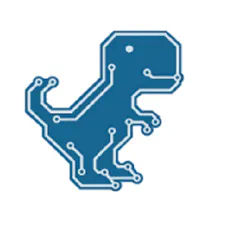# Arduino Air Quality Monitor with DSM501A Sensor

A cheap DIY air quality monitoring device.

IntermediateFull instructions provided18,546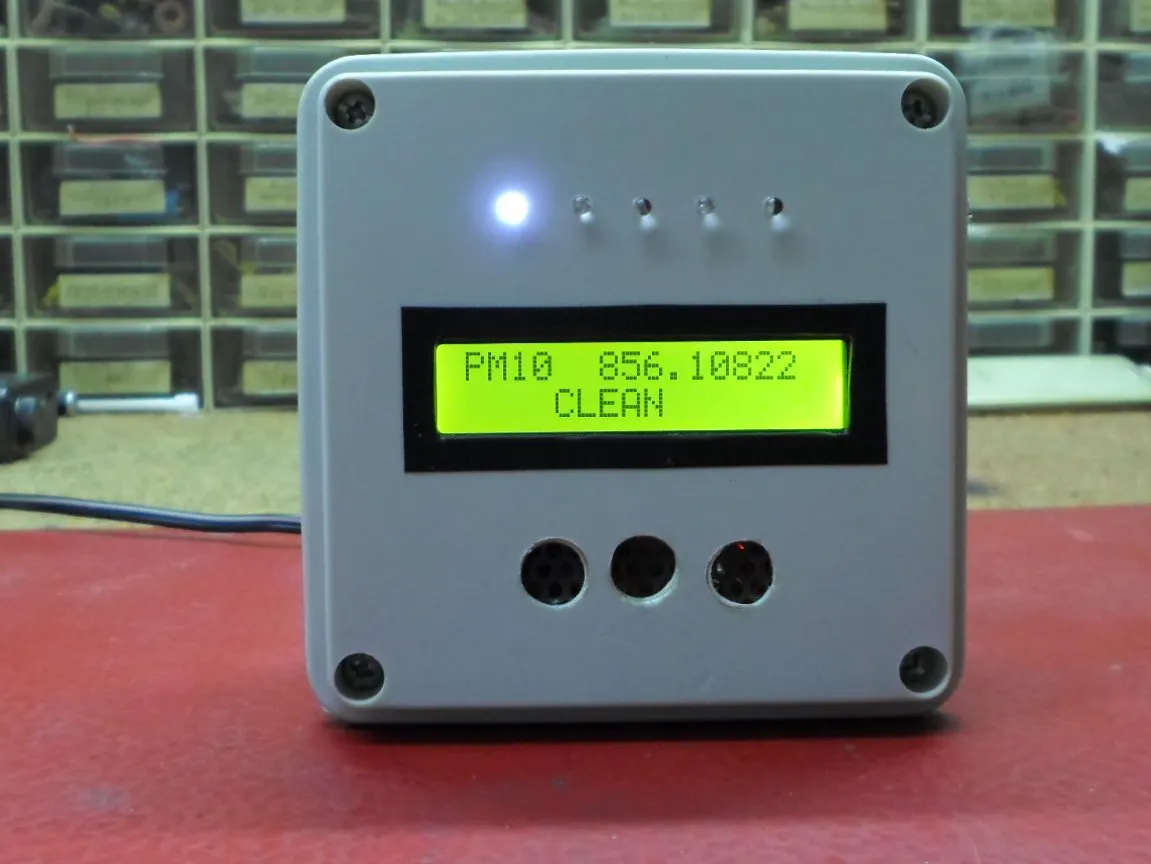## Things used in this project

### Hardware componentsArduino Nano R3
×1
 DSM501A Dust sensor module
×1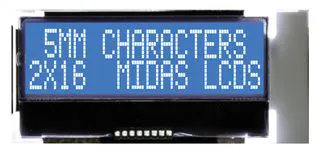Alphanumeric LCD, 16 x 2
×1
 Fan kit 40mm
×1LED (generic)
×5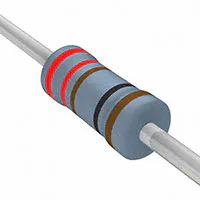Resistor 221 ohm
×1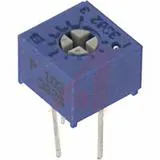Single Turn Potentiometer- 10k ohms
×1

### Software apps and online servicesArduino IDE

### Hand tools and fabrication machinesSoldering iron (generic)

## Schematics

### Schematic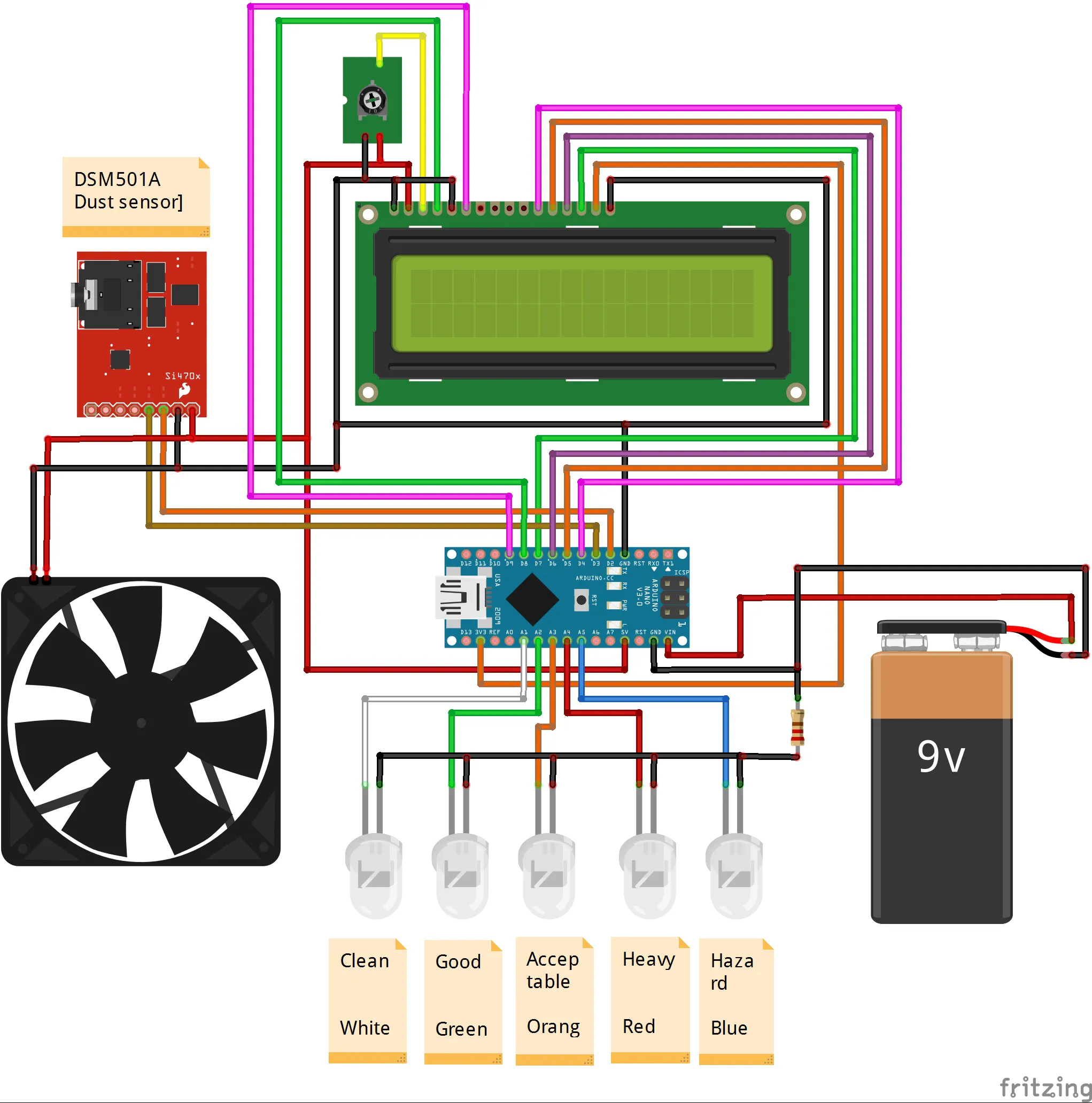## Code

### code

Arduino
``` #include <LiquidCrystal.h>
LiquidCrystal lcd(8, 9, 4, 5, 6, 7);

int pin2 = 3;
int pin1 = 2;
unsigned long duration1;
unsigned long duration2;

unsigned long starttime;
unsigned long sampletime_ms = 3000;//sampe 1s ;
unsigned long lowpulseoccupancy1 = 0;
unsigned long lowpulseoccupancy2 = 0;
float ratio1 = 0;
float ratio2 = 0;
float concentration1 = 0;
float concentration2 = 0;
int wLed = A1;
int gLed = A2;
int yLed = A3;
int rLed = A4;
int bLed = A5;

void setup() {
Serial.begin(9600);
pinMode(2,INPUT);
pinMode(3,INPUT);
pinMode(wLed,OUTPUT);
pinMode(gLed,OUTPUT);
pinMode(yLed,OUTPUT);
pinMode(rLed,OUTPUT);
pinMode(bLed,OUTPUT);
starttime = millis();//get the current time;
lcd.begin(16, 2);
}

void loop() {
duration1 = pulseIn(pin1, LOW);
duration2 = pulseIn(pin2, LOW);
lowpulseoccupancy1 = lowpulseoccupancy1+duration1;
lowpulseoccupancy2 = lowpulseoccupancy2+duration2;

if ((millis()-starttime) > sampletime_ms)//if the sampel time == 30s
{
ratio1 = lowpulseoccupancy1/(sampletime_ms*10.0);  // Integer percentage 0=>100
concentration1 = 1.1*pow(ratio1,3)-3.8*pow(ratio1,2)+520*ratio1+0.62; // using spec sheet curve

ratio2 = lowpulseoccupancy2/(sampletime_ms*10.0);  // Integer percentage 0=>100
concentration2 = 1.1*pow(ratio2,3)-3.8*pow(ratio2,2)+520*ratio2+0.62; //

lcd.setCursor(0, 0);
lcd.print("PM10 ");
lcd.setCursor(6, 0);
lcd.print(concentration1,3);

Serial.print("concentration1 = ");
Serial.print(concentration1);
Serial.print(" pcs/0.01cf  -  ");

Serial.print("concentration2 = ");
Serial.print(concentration2);
Serial.print(" pcs/0.01cf  -  ");

if (concentration1 < 1000) {
lcd.setCursor (0, 1);
for (int i = 0; i < 16; ++i)
{
lcd.write(' ');
}

lcd.setCursor(4, 1);
lcd.print("CLEAN");

digitalWrite(wLed, HIGH);
digitalWrite(gLed, LOW);
digitalWrite(yLed, LOW);
digitalWrite(rLed, LOW);
digitalWrite(bLed, LOW);
}
if (concentration1 > 1000 && concentration1 < 10000) {

lcd.setCursor (0, 1);
for (int i = 0; i < 16; ++i)
{
lcd.write(' ');
}

lcd.setCursor(4, 1);
lcd.print("GOOD");

digitalWrite(wLed, LOW);
digitalWrite(gLed, HIGH);
digitalWrite(yLed, LOW);
digitalWrite(rLed, LOW);
digitalWrite(bLed, LOW);
}

if (concentration1 > 10000 && concentration1 < 20000) {

lcd.setCursor (0, 1);
for (int i = 0; i < 16; ++i)
{
lcd.write(' ');
}

lcd.setCursor(4, 1);
lcd.print("ACCEPTABLE");
digitalWrite(wLed, LOW);
digitalWrite(gLed, LOW);
digitalWrite(yLed, HIGH);
digitalWrite(rLed, LOW);
digitalWrite(bLed, LOW);
}
if (concentration1 > 20000 && concentration1 < 50000) {
lcd.setCursor (0, 1);
for (int i = 0; i < 16; ++i)
{
lcd.write(' ');
}
lcd.setCursor(4, 1);
lcd.print("HEAVY");
digitalWrite(wLed, LOW);
digitalWrite(gLed, LOW);
digitalWrite(yLed, LOW);
digitalWrite(rLed, HIGH);
digitalWrite(bLed, LOW);
}

if (concentration1 > 50000 ) {
lcd.setCursor (0, 1);
for (int i = 0; i < 16; ++i)
{
lcd.write(' ');
}

lcd.setCursor(4, 1);
lcd.print("HAZARD");
digitalWrite(wLed, LOW);
digitalWrite(gLed, LOW);
digitalWrite(yLed, LOW);
digitalWrite(rLed, LOW);
digitalWrite(bLed, HIGH);

}

lowpulseoccupancy1 = 0;
lowpulseoccupancy2 = 0;
starttime = millis();
}
}
```

## Credits

### Mirko Pavleski

8 projects • 253 followers